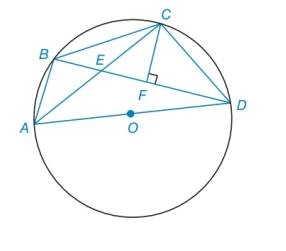Chapter 6.2, Problem 49EElementary Geometry For College St...

7th Edition
Alexander + 2 others
ISBN: 9781337614085

Solutions

Chapter
SectionElementary Geometry For College St...

7th Edition
Alexander + 2 others
ISBN: 9781337614085
Textbook Problem

In the figure, quadrilateral A B C D is inscribed in ⊙ O . Also, C F ¯ ⊥ B D ¯ .a) Explain why Δ A B E ∼ Δ D F C .b) Explain why ∠ D A B and ∠ B C D are supplementary.To determine

To explain:

The reason that ΔABEΔDFC.

Explanation

Calculation:

Given the figure,

From the figure, consider the below triangles ΔABE and ΔDFC.

To determine

To explain:

The reason that DAB and BCD are supplementary.

Still sussing out bartleby?

Check out a sample textbook solution.

See a sample solution

The Solution to Your Study Problems

Bartleby provides explanations to thousands of textbook problems written by our experts, many with advanced degrees!

Get Started

Expand each expression in Exercises 122. (4y2)y

Finite Mathematics and Applied Calculus (MindTap Course List)

Sometimes, Always, or Never: If c is a critical number, then f′(c) = 0.

Study Guide for Stewart's Single Variable Calculus: Early Transcendentals, 8th

True or False: If and , then .

Study Guide for Stewart's Multivariable Calculus, 8th